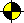### Guide to Prolog Programming © Roman Barták, 1998 Home Prolog in Examples Prolog Data Structures Graphs in Prolog Previous | Contents | Next Generalized Algorithm for Graph Search[kernel] [coloring] [Dijkstra]

In this subsection we present a general schema of algorithm for searching graphs. This schema is based on notions of open and closed vertices. The open vertex was visited by the algorithm but it has not been explored/processed yet, while the closed vertex has already been visited and explored.

The algorithm takes some open vertex V and expands it, i.e., the algorithm process the vertex V, finds its neigbourhood, joins this neigbourhood with the rest of open vertices and add this vertex V to the set of closed vertices. Note, that closed vertices are removed from the neigbourhood before joining with open vertices. The algorithm stops as soon as the set of open vertices is empty.

```% open_close_search(+Graph,+Open,+Closed,-Result)
open_close_search(Graph,[],Closed,Result):-
tune_result(Graph,Closed,Result).
open_close_search(Graph,[Vertex|Open],Closed,Result):-
explore(Vertex,Graph,Neighbourhood,ClosedVertex),
diff(Neighbourhood,Closed,OpenNB), % remove closed vertices
merge(OpenNB,Open,NewOpen),        % join with rest open vertices
open_close_search(Graph,NewOpen,[ClosedVertex|Closed],Result).```

The above program contains "hooks", e.g., tune_result or explore, which have to be programed to get a particular algorithm. We show such extensions now (procedures for hooks are labeled by bold text).

###Coloring

First, we program the graph coloring algorithm using the above presented general schema. This algorithm corresponds to the well known algorithm that colors one vertex and, then, it colors the vertex's neighbourhood and so on. Note, that after coloring one graph segment, we have to restart the search in tune_result procedure if there remains other components.

```% open_close_coloring(+Graph,+Colors,-Coloring)
open_close_coloring(Graph,Colors,Coloring):-
vertices(Graph,[V|Vertices]),
open_close_search(Graph-Colors,[V-Colors],[],Coloring).

explore(V-Cs,Graph-Colors,Neighbourhood,V-C):-
member(C,Cs),                 % assign color to the vertex
neighbourhood(Graph,V,NB),    % find neigbourhood
delete(C,Colors,NBColors),     % prepare possible colors for neighbourhood
add_colors(NB,NBColors,Neigbourhood). % assign colors to neighbourhood

diff([],_,[]).
diff([V-Cs|CVs],Closed,NonClosed):-
(member(V-_,Closed) -> NonClosed=[V-Cs|Rest] ; NonClosed=Rest),
diff(CVs,Closed,Rest).

merge([],Open,[]).
merge([V-Cs|CVs],Open,[V-NCs|Rest]):-
(member(V-OCs,Open)
-> intersection(Cs,OCs,NCs)   % classical set intersection
;  NCs=Cs),
NCs\=[],                         % it is possible to assign color
merge(CVs,Open,Rest).

tune_result(Graph-Colors,Closed,Result):-
vertices(Graph,Vertices),
diff(CVertices,Closed,NonClosed),
(NonClosed=[CV|_]                % is there other graph component?
-> open_close_search(Graph-Colors,[CV],Closed,Result)
;  Result=Closed).```

###Dijkstra's algorithm

Now, we program the extension of the above open/closed schema that behaves like Dijkstra's algorithm which uses sets of open and closed vertices naturally. Remind, that Dijkstra's algorithm finds minimal distance to all vertices in the graph from given vertex.

```% open_close_dijkstra(+Graph,+Start,-MinDist)
open_close_dijkstra(Graph,Start,MinDist):-
open_close_search(Graph,[Start-0],[],MinDist).

explore(V-D,Graph,Neigbourhood,V-D):-
neighbourhood(Graph,V,NB),

VD is D+D1,

diff([],_,[]).
diff([V-D|VDs],Closed,NotClosed):-
(member(V-_,Closed) -> NotClosed=[V-D|Rest] ; NotClosed=Rest),
diff(VDs,Closed,Rest).

merge([],Open,Open).
merge([V-D1|VDs],Open,NewOpen):-
(del(V-D2,Open,RestOpen)
-> min(D1,D2,D),ins(V-D,RestOpen,SOpen)
;  ins(V-D1,Open,SOpen),
merge(VDs,SOpen,NewOpen).

del(X,[X|T],T).
del(X,[Y|T],Rest):-X\=Y,del(X,T,Rest).

ins(VD,[],[VD]).
ins(V-D,[U-D1|T],[V-D,U-D1|T]):-D<=D1.
ins(V-D,[U-D1|T],[U-D1|Rest]):-D>D1,ins(V-D,T,Rest).

tune_result(_,Closed,Closed).```

Generalization can simplify the program development and understanding.

[kernel] [coloring] [Dijkstra]